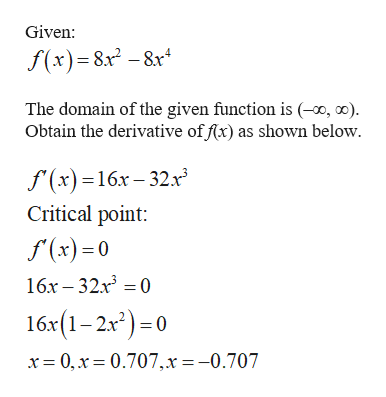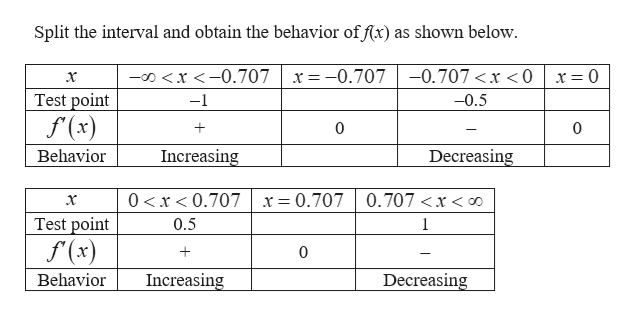# Let f(x)=8x^2−8x^4\. Find the open intervals on which f is increasing (decreasing). Then determine the xx-coordinates of all relative maxima (minima). 1.  f is increasing on the intervals 2.  f is decreasing on the intervals 3.  The relative maxima of f occur at x = 4.  The relative minima of f occur at x =

Question
18 views

Let f(x)=8x^2−8x^4\. Find the open intervals on which f is increasing (decreasing). Then determine the xx-coordinates of all relative maxima (minima).

 1 f is increasing on the intervals 2 f is decreasing on the intervals 3 The relative maxima of f occur at x = 4 The relative minima of f occur at x =
check_circle

Step 1help_outlineImage TranscriptioncloseGiven: f(x)=8x2-8r The domain of the given function is (-0, ) Obtain the derivative of f(x) as shown below f(x)16x-32x Critical point: f(x)0 16x-32x 0 16x(1-2x2)=0 x 0,x 0.707,x=-0.707 fullscreen
Step 2help_outlineImage TranscriptioncloseSplit the interval and obtain the behavior of f(x) as shown below. ox-0.707 x -0.707 -0.707

### Want to see the full answer?

See Solution

#### Want to see this answer and more?

Solutions are written by subject experts who are available 24/7. Questions are typically answered within 1 hour.*

See Solution
*Response times may vary by subject and question.
Tagged in

### Other